Since R2023b

## Description

The `MeshReader` object stores the properties of the mesh such as the points, triangles, and tetrahedra in the mesh, edge lengths of the triangles, growth rate, and mesh quality. You can use this information to fine tune the mesh for your application. You can also visualize the mesh of the metal region, dielectric region, or the entire antenna or array structure and detect bad features in the mesh.

## Creation

You can create the `MeshReader` object using the `mesh` function. There are two ways to create this object:

• Run any port, surface, or field analysis on the antenna or array to automatically generate the mesh. Then use `m = mesh(object)` syntax to create the `MeshReader` object.

• Manually mesh the antenna or array by setting `MaxEdgeLength` property of the mesh to create the `MeshReader` object. For example, `m = mesh(object,MaxEdgeLength=0.01)` creates a `MeshReader` object.

## Properties

expand all

Cartesian coordinates of points in mesh, returned as a 3-by-N matrix double. N represents the number of points and each column of the matrix represents x, y, and z-coordinates of the points.

Example: `[-0.0188 -1e-3 0; -0.0375 -0.0188 0]`

Data Types: `double`

Mesh triangles connectivity list, specified as a 4-by-N integer matrix, where N is the number of triangles in the mesh. First three rows of each column in `Triangles` contains the vertex IDs that define a triangle. The vertex IDs are the column numbers of the `Points` property. The ID of a triangle in the mesh is the corresponding column number in the `Triangles` property.

Example: `[96 97 146 1;...;1 293 294 2]`

Data Types: `double`

Mesh tetrahedra connectivity list, specified as a 4-by-N integer matrix, where N is the number of tetrahedra in the mesh. Each column of `Tetrahedra` contains the vertex IDs that define a tetrahedron. The vertex IDs are the column numbers of the `Points` property. The ID of a tetrahedron in the mesh is the corresponding column number in the `Tetrahedra` property. This property has an empty value for the antennas without a dielectric substrate.

Example: `[96 97 146 438;...;12 547 305 304]`

Data Types: `double`

Maximum edge length of triangles in mesh, returned as a scalar in meters.

Example: `0.01`

Data Types: `double`

Smallest edge length of the triangles in the mesh, returned as a positive scalar in meters.

Example: `2e-3`

Data Types: `double`

Gradation in the triangle sizes of the mesh, returned as a scalar in the range (0,1).

Example: `GrowthRate=0.7` states that the growth rate of the mesh is 70 percent.

Data Types: `double`

Mesh quality is a measure of degree of structural similarity of the mesh triangles to an equilateral triangle. `MinimumMeshQuality` is the lowest acceptable mesh quality out of all the mesh triangles, returned as a scalar in the range (0,1). A minimum mesh quality of `1` means all the mesh triangles are equilateral.

Example: `MinimumMeshQuality=0.72` states that the lowest quality triangle in the mesh is 72 percent similar to an equilateral triangle.

Data Types: `double`

Mode of mesh creation, returned as either `"auto"` or `"manual"`. The `MeshMode` is `"auto"` when the mesh gets created due to running any analysis function on the antenna or array. The `MeshMode` is `"manual"` when the mesh is created by specifying the `MaxEdgeLength` value in the `mesh` function run on an antenna, array, or custom geometry.

Example: `MeshMode="manual"`

Data Types: `string`

## Object Functions

 `showDielectricSurfaceMesh` Display dielectric surface mesh of antenna or array `showDielectricVolumeMesh` Display dielectric volume mesh of antenna or array `showMeshAll` Display complete metal-dielectric mesh of antenna or array `showMetalMesh` Display metal mesh of antenna or array

## Examples

collapse all

This example shows how to read mesh parameters of a microstrip patch antenna and view the metal, dielectric, and complete mesh.

Create Microstrip Patch Antenna

Create a microstrip patch antenna with copper conductor and FR4 PCB substrate. Run impedance analysis on this antenna at 1.67 GHz to automatically generate the mesh.

```p = patchMicrostrip(Conductor=metal("Copper"),Substrate=dielectric("FR4")); figure impedance(p,1.67e9);```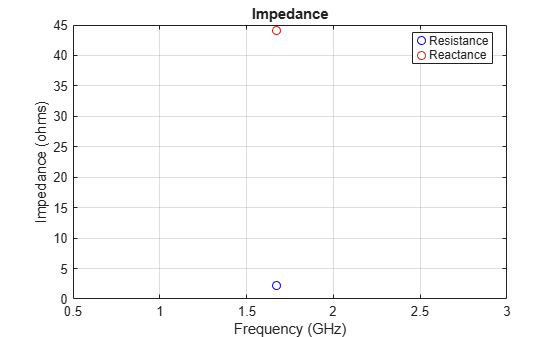Read the mesh parameters such as number of points, triangles, and terahedra, maximum and minimum edge length, mesh growth rate, and quality using the `MeshReader` object.

`m = mesh(p)`
```m = MeshReader with properties: Points: [3x1644 double] Triangles: [4x2014 double] Tetrahedra: [4x4620 double] MaxEdgeLength: 0.0044 MinEdgeLength: 2.2012e-04 GrowthRate: 0.9500 MinimumMeshQuality: 0.0803 MeshMode: 'auto' ```

View Mesh of Antenna

View the metal mesh.

```figure showMetalMesh(m)```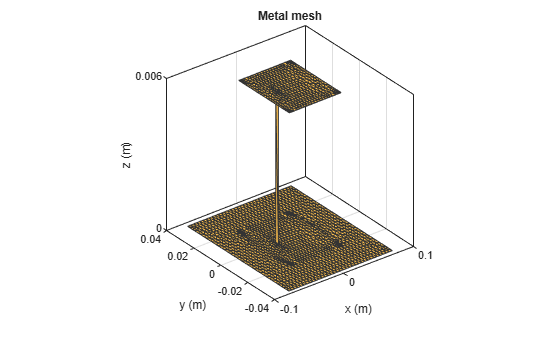View the dielectric surface mesh.

```figure showDielectricSurfaceMesh(m)```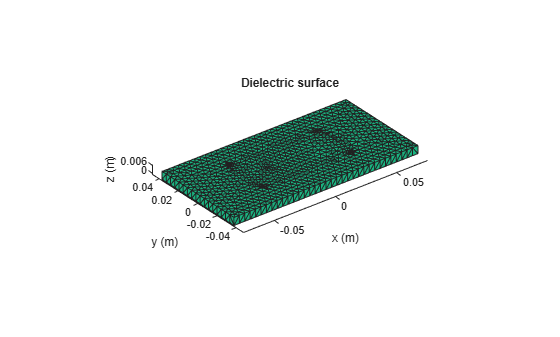View the dielectric volume mesh.

```figure showDielectricVolumeMesh(m)```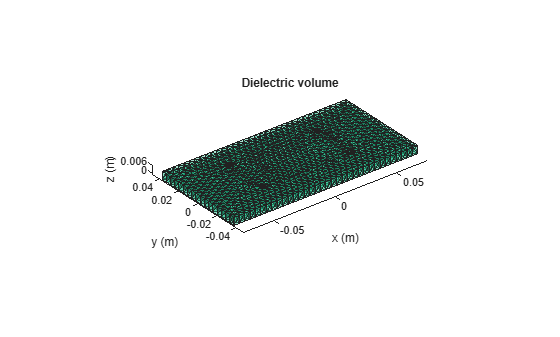View the overall mesh.

```figure showMeshAll(m)```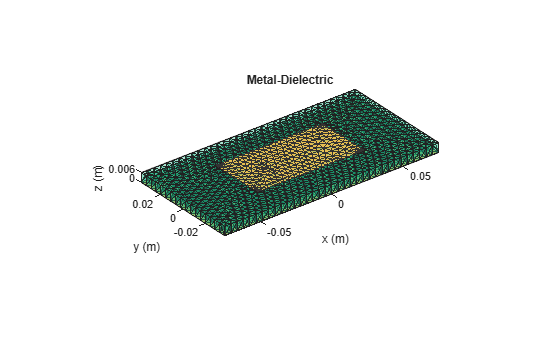## Tips

The `MeshReader` object stores the `triangulation` information of the mesh in the form of points and triangles connectivity list. This allows `stlFileChecker` functions to be directly used on the `MeshReader` object to detect and display bad features of the mesh. For example, below code shows the detection and display of T-Vertices in the mesh of a microstrip patch antenna operating at 1.67 GHz.

```ant = patchMicrostrip(Conductor="Copper", Substrate=dielectric("FR4")); impedance(ant,1.67e9); m = mesh(ant) detectTVertices(m); m.TVertices showTVertices(m)```

Alternatively, you can write the mesh data to a STL file using `stlwrite` function and then run `stlFileChecker` on that STL file to detect bad features of the mesh. For example, below code shows the detection of all the bad features in the mesh by converting the mesh data to a STL file using `stlwrite` function and running `stlFileChecker` on that data. Once all the bad features are detected, you can choose individual feature to be highlighted on the mesh plot using functions of the `stlFileChecker` object.

```ant = patchMicrostrip(Conductor=metal("Copper"),... Substrate=dielectric("FR4")); impedance(ant,1.67e9); m = mesh(ant) tr = triangulation(m.Triangles(1:3,:)',... m.Points(:,1:max(unique(m.Triangles(1:3,:)))')'); stlwrite(tr,"sampleFile.stl"); stlFileChecker("sampleFile.stl")```

## Version History

Introduced in R2023b